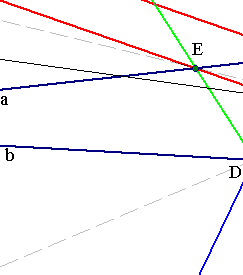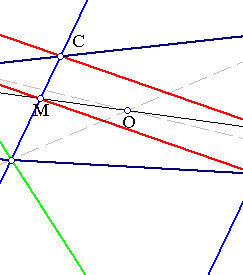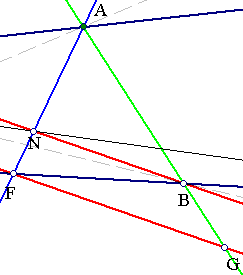##Pappus theorem, a special case

Consider three points {A,C,E} on line a and three points {B,F,D} on line b and join them as shown. Assume further that the line pair {ED,AB} consists of parallels (green lines) and the same is true for the line pair {DC,FA} (blue lines). Then the third line pair {BC,EF} consists also of parallels (red lines).
In addition the intersection-points of the corresponding line pairs M(EF,DC), O(DA,EB), N(AF,BC) are collinear (this is a special case of Pappus theorem).To prove the first claim consider:
Line-bundle at E: EC/CA= ED/DK because of blue parallels.
Line-bundle at F: BG/BA = ED/DK because of green parallels.
Hence from the parallelity of blue line-pair and green line-pair follows the parallelity of the red line-pair.

The intersection point O of the line pair {AD,EB} divides AD in ratio ED/AB, since triangles {DEO,ABO} are similar.
The intersection point O' of the line pair {AD,MN} divides AD in ratio DM/AN, since triangles {DO'M,AO'N} are similar.
But ED/AB = DM/AN since triangles {EMD,BNA} are also similar. Hence O coincides with O'. This shows the collinearity of the three points.

Remark We obtain easily a proof of the general case of Pappus theorem by reducing it to this one. For this it suffices to apply to the general case a projectivity F mapping the intersection points of line pairs {ED,AB} and {DC,FA} to two points at infinity. The resulting figure is similar to the above and the collinearity property is preserved by projectivities. Hence by applying F-1 we obtain the proof of the general case.